# 【杂文】我们都有光明的前途（回忆录）

### 【上高中以前的事】

“初中留在了本地，那么高中就一定要把孩子送出去读书，否则前途渺茫。”

（其实我一直有怀疑他们俩是不是来自某个大家族，归隐小县城躲避仇家。家里有钱、在大城市有房子、父母有见识，却偏要跑到这个地方来上中学。按照他们的说法，是因为其中一方父母的户口在此，到底是否如此也就不得而知了）

“反正不管到了哪座学校都得靠自己，去了好学校学轻松一点，去了坏学校学辛苦一点，最后的结果都一样。我愿意吃一点苦。”

“我们家准备了这么久，高中的入学费、生活费也都拼命挣钱攒了出来，就这样突然放弃........”

“男孩子，就应该要出去闯，不怕闯。”

### 【我的 OI 生涯经历】

$$\text{NOIP 2018}$$ 前有一周多的停课，那时候教练给我们强行灌输了最短路算法、树状数组等等一堆东西，但最后考场也就只用到一个最短路，甚至还是不带链表的版本（当时忘了怎么写了.....）。$$\text{NOIP}$$ 回来后那几个月往死里学 $$\text{whk}$$，最后也是成功翻了身。

$$378$$$$\text{zth}$$ $$373$$$$\text{yms}$$ $$368$$ 。那时我们仨之间几乎不存在差距。甚至于我和 $$\text{yms}$$ 考场上的骗分心路历程和挂分处都一样，只是他 $$\text{day1}$$ 比我少写了 $$T3$$$$10pts$$ 爆捜（好像是说看分太少不想写来着）。

$$\text{zth}$$ 几乎不会主动去找题、刷题。

$$\text{yms}$$ 其实是因为动摇了（大概是因为 $$\text{-OH}$$ 的原因吧）。

$$(1).$$ 花高一、高二一年多的时间打基础，然后依靠短短几个月学习省选及以上的内容，和来自各个地方的强者争省队名额。

$$(2).$$ 在初中甚至小学打好基础，依靠高一、高二一年多的时间锻炼脑子。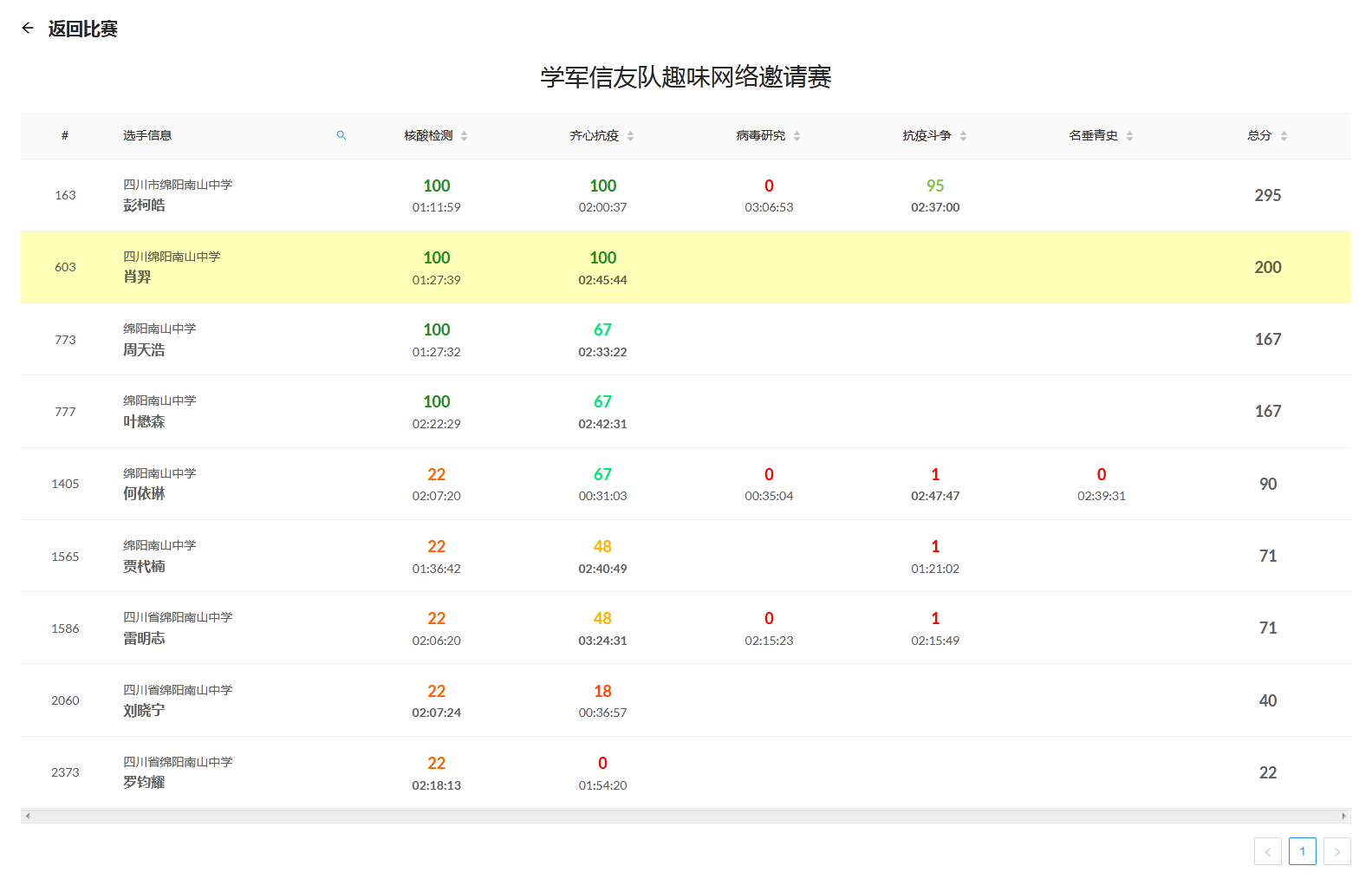（另：注意到 “四川市” 了吗？）

（感觉窝打 $$\text{NOIO}$$ 使用到的算法是最多的？大考基本都是靠暴力在混诶....）

$$5$$$$12$$ 日，学习时间安排变动，这使我意识到事情的严重性，并开始反思自己最近一段时间的学习状态。

$$\text{SCOI}$$ 被改成了一试三题，这让我感到非常的不爽。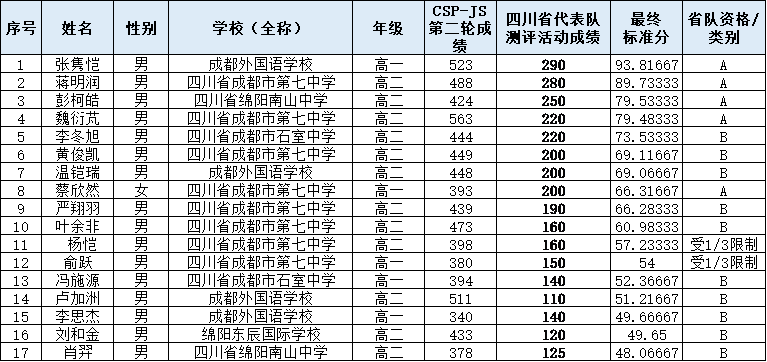$$\text{pkh}$$ 则始终是一座无法逾越的高山、一颗天边闪耀的明星，一边在赛场崭露锋芒，一边给我以前进的方向。

Q: $$\text{WC}$$ 听不懂/不想听怎么办？
A: 水群。

$$\text{WC}$$ 最后还邀请到了曾经的 $$\text{NOI Cu OIer}$$、现 $$\text{Google}$$ 慕尼黑软件工程师钟诚来进行讲座。

“一名普通 $$\text{NOI}$$ 选手”，这一描述仿佛就在形容我自己。

$$\text{A}$$ $$\text{Au}$$ $$\text{OI}$$集训队，
$$\text{W}$$ 裸考 $$\text{whk}$$ 走清北。

$$\text{WC 2020}$$ 仍是全打了暴力，$$\text{rk 97}$$$$\text{Ag}$$

$$\text{APIO 2020}$$ 炸得连灰都不剩，但毕竟不是什么重要比赛，只不过会遗憾少拿了块牌，对我的心态也没产生啥影响。

$$\text{updata }2020.8.24:$$

### 【获奖证明】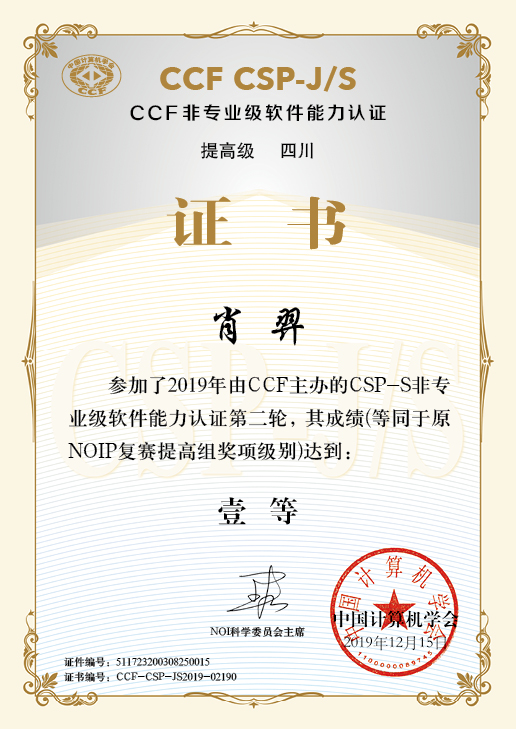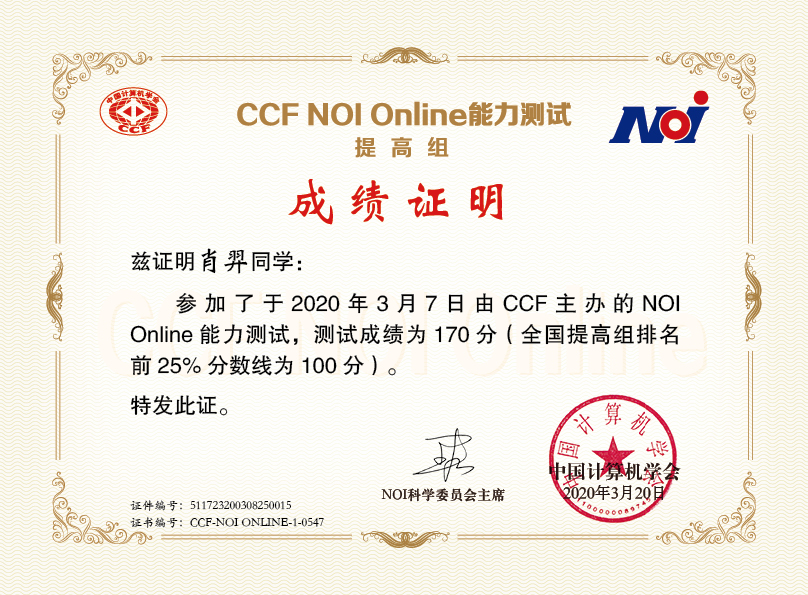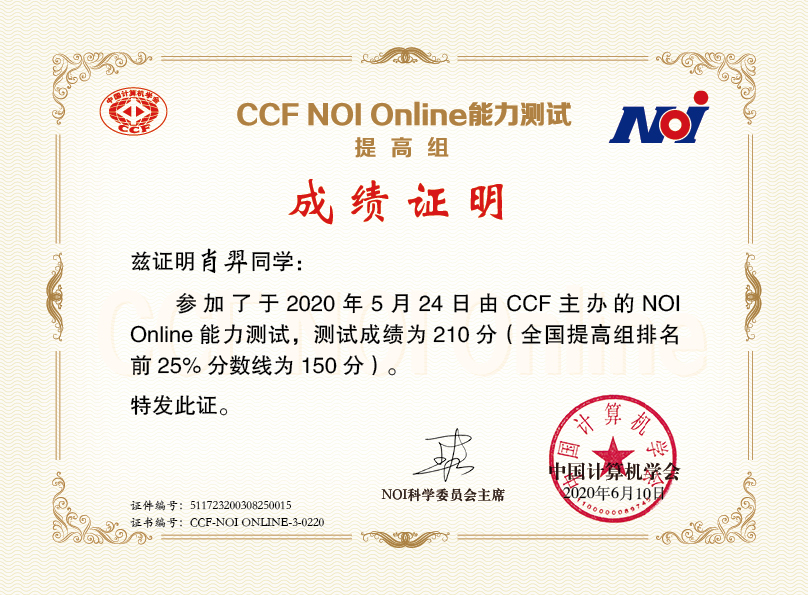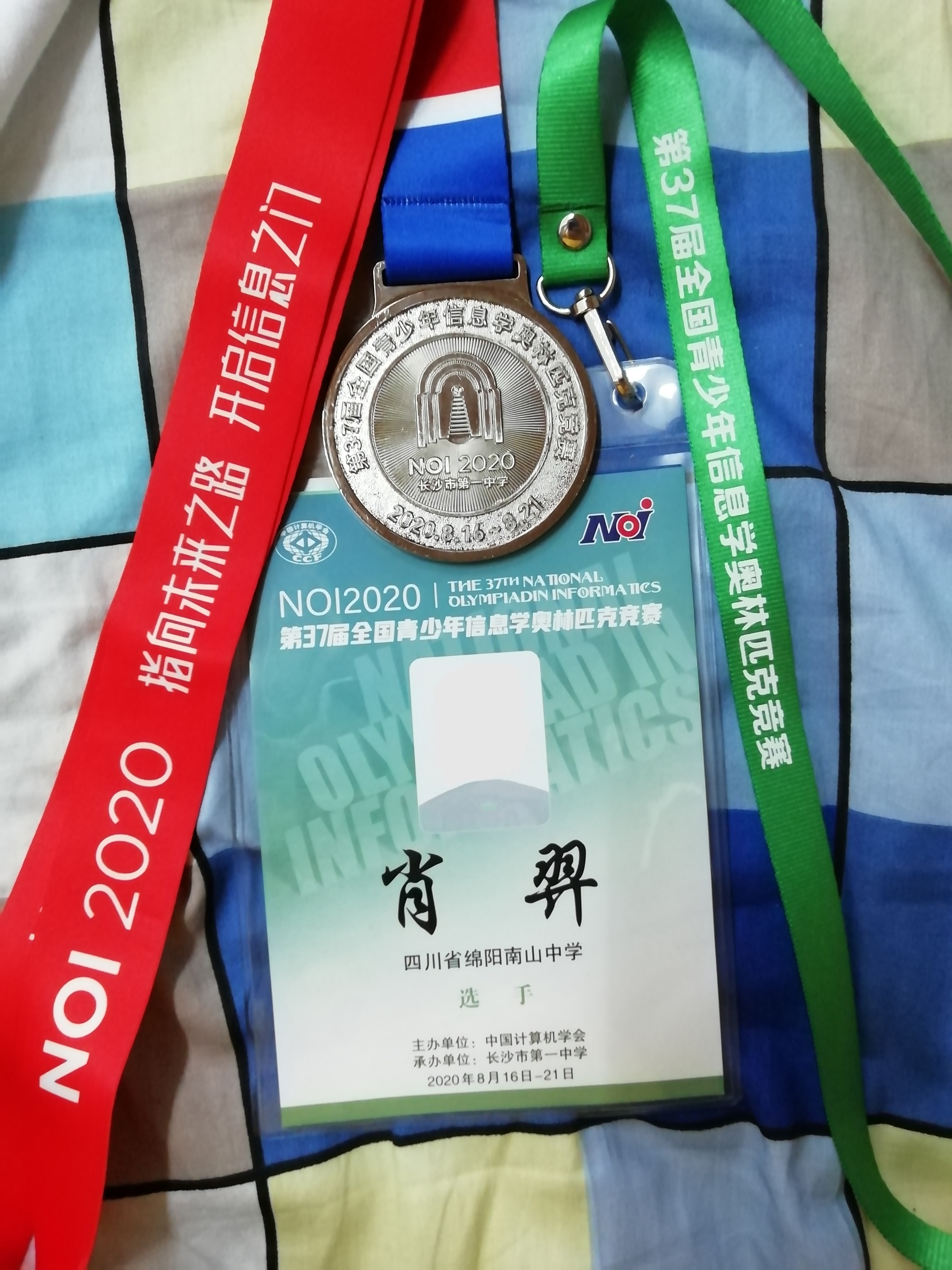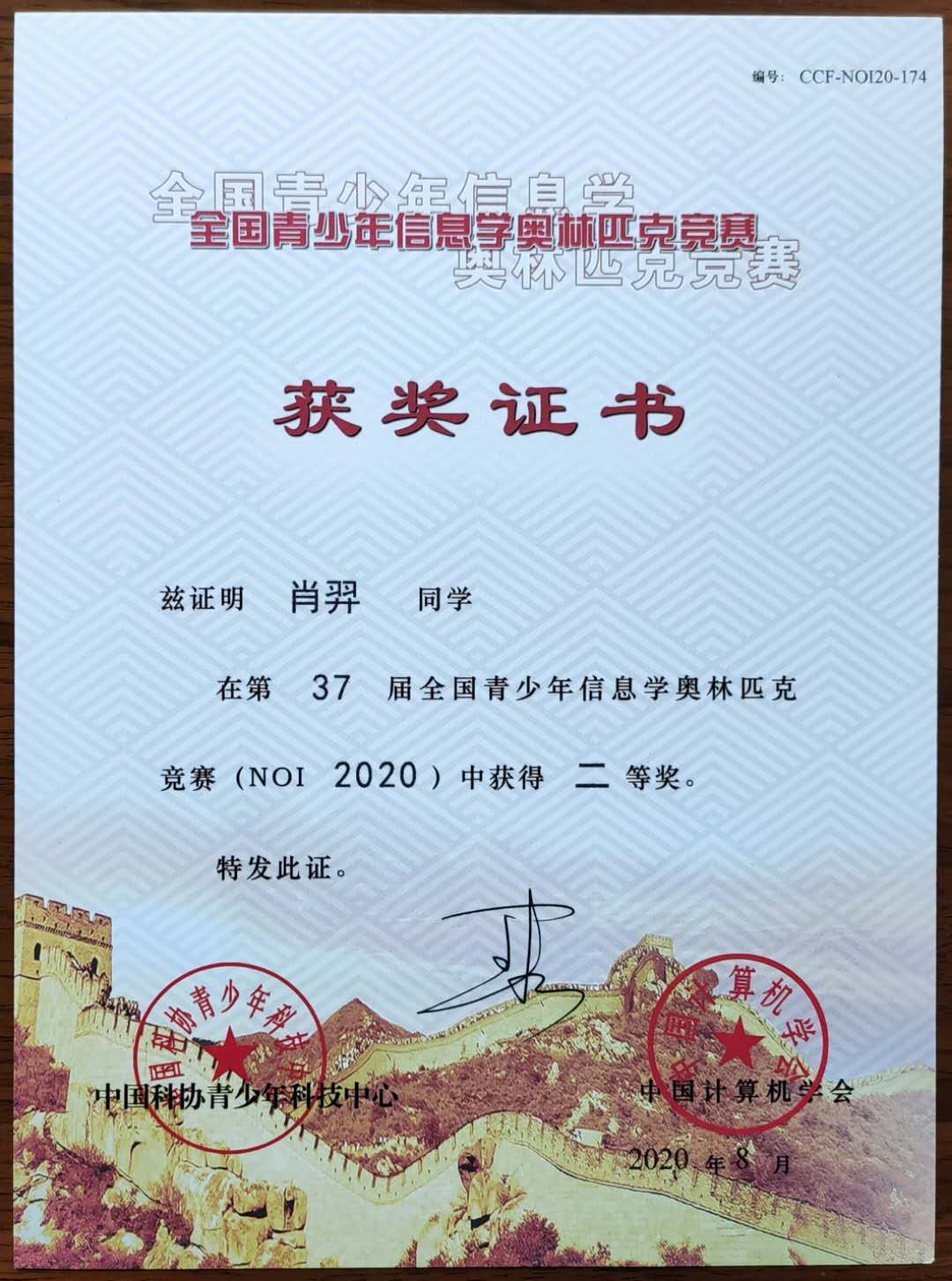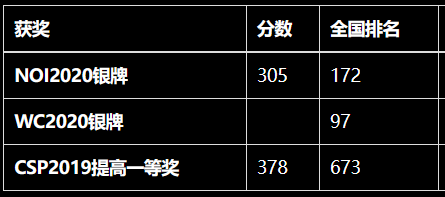### 【一些杂乱的想法和回忆】

（什么时候想起来了大概还会补充）

• $$\text{yqg}$$ 学长一直是我仰慕的对象。他也是从高中开始才接触到 $$\text{OI}$$，但他依靠自身努力拿到了 $$\text{Ag}$$，排名 $$76$$ 。我会从他的刷题记录中随机挑选一些来做，$$\text{SCOI}$$$$\text{NOI}$$ 以他的分数作为目标
，那两篇游记我看了无数次，也时常思考：如果以我现在的水平，能不能做出那些他切掉的题目？能不能得到同样的分数？

• 几天后的 $$8$$$$25$$ 日是我 $$17$$ 岁生日（阳历），而今年的银牌就是最好的生日礼物。

• 疫情期间抽时间学习 $$\text{EasyX}$$，开坑了一个小工程，码了上千行，不过后来咕掉了。高三 $$\text{whk}$$ 之余可能会抽周末时间继续写下去。【开发进度】

• 我喜欢填补一些算法上的空白，比如对点分树的各种套路进行总结【链接】，详细讲述广义 $$\text{SAM}$$ 正确写法并对各类“盗版”写法的复杂度及 $$\text{hack}$$ 方案进行研究【链接】，这会使我感觉到自己是在为 $$\text{OI}$$ 界做出贡献。
我似乎从小就有着收藏癖，喜欢分类归纳、整理、列表、列树、列目录，因此我整理了计算几何全家桶【链接】、算法全套模板【链接】、全套 $$\text{dp}$$ 优化方案【链接】、各类数论数学知识点分类汇总【链接】

——【杂文】$$\text{NOIP2018}$$ 蒟蒻自闭记

——【杂文】$$\text{CSP2019}$$ 蒟蒻 $$\text{AFO}$$ (假)记

“我想进省队，拿银牌、金牌，我想在高中阶段留下一个能让我为之骄傲一生的东西。”

“我喜欢音乐，尤其是钢琴曲，我想学钢琴。”

“我想画画，学分镜，学写故事，学人物心理，然后画一本漫画，描绘我所向往的生活。”

“我想买一套喜欢的轻小说，支持作者。”

......
——【杂文】记我高中生涯的第二次转折

——【杂文】$$\text{SCOI2020}$$ 游记

——あさがおと加瀬さん【杂文】银色的 $$\text{NOI2020}$$（退役记）

——$$\text{OI Diary-Madia Lemon}$$

$\color{red}{\Large{\textbf{愿我们都能有光明的前途！}}}$

posted @ 2020-08-22 20:09  辰星凌  阅读(2894)  评论(5编辑  收藏  举报